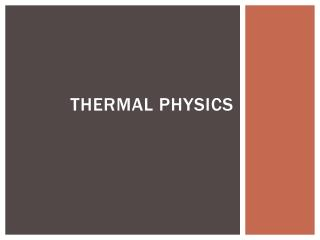Download PresentationThermal Physics

# Thermal Physics - PowerPoint PPT PresentationDownload Presentation## Thermal Physics

- - - - - - - - - - - - - - - - - - - - - - - - - - - E N D - - - - - - - - - - - - - - - - - - - - - - - - - - -
##### Presentation Transcript

1. Thermal Physics

2. States of matter • Matter is most commonly found in solid, liquid or gas form. We will discuss the properties of these different states of matter.

3. Solids • Solids have a fixed shape and a fixed volume. • The molecules in a solid have a rigid structure. • The force of attraction between molecules is strong.

4. Liquids • Liquids have a fixed volume but change shape to fit the container. • The molecules in a liquid stay in contact but move around freely. • The force of attraction is strong enough to keep the molecules from completely moving away.

5. gases • Gases do not have a fixed volume or shape. • The molecules in a gas are far apart and move quickly. • The forces of attraction between molecules is negligible.

6. Temperature in gases • The temperature of a gas is the average kinetic energy of its molecules. • Objects with a high temperature have molecules moving at a high speed. If the temperature is decreased the speed of the molecules decreases.

7. Pressure in a gas • The pressure of a gas on a surface is due to the impacts of gas molecules with the surface. • When a molecule impacts the surface it exerts a tiny force. Billions of these impacts occur every second creating a steady pressure on the surface.

8. Changes of state • The following diagram shows the names of the different changes of state.

9. Changes of state • The changes of state can be described by the movement of molecules. • Melting – when a solid is heated the molecules begin vibrating to the point at which they break free of the rigid structure. • Freezing – When a liquid is cooled the molecules slow down and form a rigid structure.

10. Changes of state • The changes of state can be described by the movement of molecules. • Boiling – When a liquid is heated the molecules move quickly and break free from each other. • Condensing – When a gas is cooled the molecules move more slowly and the force of attraction increases.

11. Summary • Molecules in a solid are in a fixed structure. • Molecules in a liquid move in contact with each other. • Molecules in a gas are far apart and move at high speed. • Increasing the temperature of a gas increases the average speed of its molecules. • The pressure of the gas on a surface is caused by its molecules repeatedly hitting the surface. • Practice: pg 71 #1,2 and pg 73 #1,2

12. Pressure, temperature and volume in gases

13. Random motion of particles • In 1785, Robert Brown observed pollen grains floating on water. He observed that the pollen grains moved randomly. • Using molecular theory, it has been explained that the small water molecules were constantly colliding with the large pollen grain. This caused it to move randomly. • This motion is called Brownian Motion.

14. Pressure and temperature • If we had a container of sealed gas, what would happen to the pressure if we increase the temperature? (Note: we are keeping the same volume.) • The pressure would increase because the molecules would be moving faster and there would be more collision with the walls of the container.

15. Gas pressure and volume • Imagine a piston filled with a gas. • The temperature and mass of the gas are constant. • If we force the piston down, what will happen to the pressure?

16. Gas pressure and volume • If the piston is forced down, the volume decreases. • The pressure in the tube will increase because the molecules will impact the surface more often.

17. Gas pressure and volume • What would happen to the piston if we lift the piston upwards?

18. Gas pressure and volume • If the piston is forced up, the volume increases. • The pressure in the tube will decrease because the molecules will impact the surface less often.

19. Gas pressure and volume • We see that when volume decreases the pressure increases. When the volume increases the pressure decreases. • This means that volume and pressure are “inversely proportional”.

20. Volume and pressure • Pressure • Symbol – P • Unit – Pascal • Unit Symbol – Pa • Volume • Symbol – V • Unit – meter cubed or centimeter cubed • Unit Symbol – m3 or cm3

21. Boyle’s Law • For a fixed mass of gas at a constant temperature: pressure x volume = constant • When you are comparing two situations you can use the following equation: P1V1 = P2V2

22. Boyle’s law example • A fixed mass of gas has an initial volume of 15cm3. When the volume is increased to 45cm3 the pressure is measured at 60kPa. What was the original pressure?

23. Summary • Brownian motion is the random motion or small particles due to the impacts of gas molecules on each particle. • The pressure of a gas in a sealed container increases if the gas temperature is increased. • For a fixed mass of gas at constant temperature, the pressure x volume = constant.